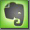International Letter Code - Radio Communication - ITU, NATO, ICAO

A = Alpha
Ä = Alpha-Eche B = Bravo
C = Charlie
D = Delta
E = Echo
F = Foxtrott
G = Golf
H = Hotel
I = India
J = Juliet
K = Kilo
L = Lima
M = Mike
N = November
O = Oskar
Ö = Oscar Echo P = Papa
Q = Quebec
R = Romeo
S = Sierra
T = Tango
U = Uniform
Ü = Uniform Echo V = Viktor
W = Whiskey
X = X-Ray
Y = Yankee
Z = Zulu